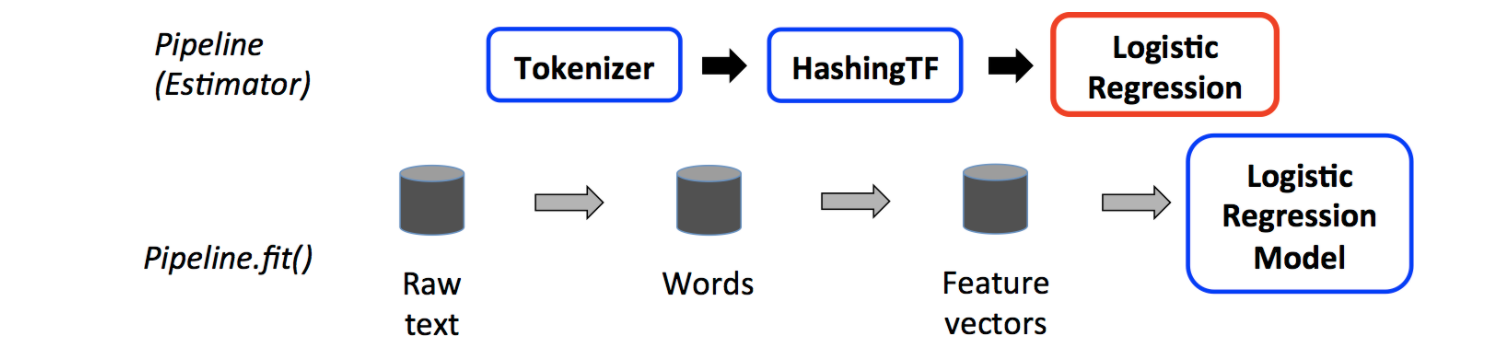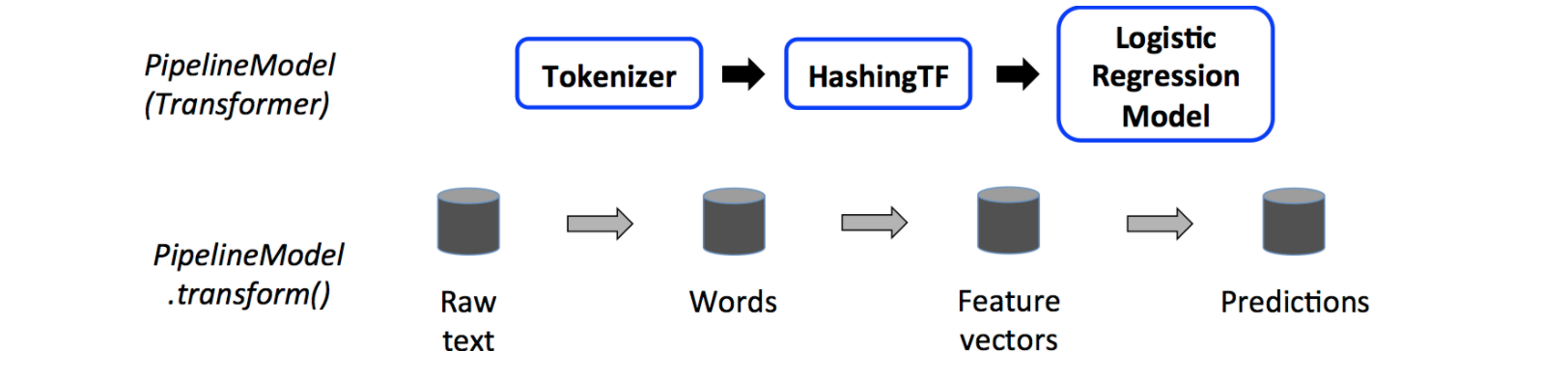# ML管道

## 管道的主要概念

MLlib对用于机器学习算法的API进行了标准化，从而使将多种算法组合到单个管道或工作流中变得更加容易。
-DataFrame：此ML API使用DataFrameSpark SQL作为ML数据集，可以保存各种数据类型。例如，一个DataFrame可能有不同的列，用于存储文本，特征向量，真实标签和预测。

• Transformer：一个Transformer是一种算法，其可以将一个DataFrame到另一个DataFrame。例如，ML模型是一种Transformer将DataFrame具有特征的a转换为DataFrame具有预测的a的模型。

• Estimator：An Estimator是一种算法，可以适合DataFrame产生Transformer。例如，学习算法是在上Estimator进行训练DataFrame并生成模型的算法。

• Pipeline：将Pipeline多个Transformer和链接Estimator在一起以指定ML工作流程。

• Parameter：所有Transformer和Estimator现在共享一个用于指定参数的通用API。

## DataFrame

Machine learning可以应用于多种数据类型，例如矢量，文本，图像和结构化数据。该API采用Spark SQL中的DataFrame，以支持多种数据类型。

DataFrame支持许多基本类型和结构化类型。请参考Spark SQL数据类型参。除了Spark SQL指南中列出的类型之外，DataFrame还可使用ML Vector类型。

## Pipeline components(管道组件)

### Transformers(转换器)

Transformers是一种抽象，其中包括特征转换器和学习的模型。从技术上讲，Transformer实现了transform()方法，该方法通常通过附加一个或多个列将一个DataFrame转换为另一个DataFrame。例如：

• 特征转换器可以获取一个DataFrame，读取一列（例如：文本），将其映射到一个新列（例如：特征向量），然后输出一个新的DataFrame并附加映射的列。

• 学习模型可能需要一个DataFrame，读取包含特征向量的列，预测每个特征向量的标签，然后输出带有预测标签的新DataFrame作为列添加。

### Properties of pipeline components(管道组件属性)

Transformer.transform()和Estimator.fit()都是无状态的。将来，可通过替代概念来支持有状态算法。

## Pipeline(管道)

1. 将每个文档的文本拆分为单词。
2. 将每个文档的单词转换成数字特征向量。
3. 使用特征向量和标签学习预测模型。

MLlib将这样的工作流表示为“Pipeline”，它由要按特定顺序运行的一系列PipelineStagesTransformer和Estimator）组成。

### 工作流程

Pipeline被指定为阶段序列，每个阶段可以是一个TransformerEstimator。这些阶段按顺序运行，并且输入DataFrame在通过每个阶段时都会进行转换。对于Transformer阶段，在DataFrame上调用transform()方法。对于Estimator阶段，调用fit()方法以生成一个Transformer（它将成为PipelineModel或已拟合Pipeline的一部分），并且在DataFrame上调用该Transformer的transform()方法。Pipelines 和 PipelineModels有助于确保训练和测试数据经过相同的特征处理步骤。

### 详细

• DAG Pipeline：Pipeline的阶段被指定为有序数组。此处给出的所有示例均适用于线性管道，即每个阶段使用前一阶段产生的数据的管道。只要数据流图形成有向无环图（DAG），就可以创建非线性管道。当前基于每个阶段的输入和输出列名称（通常指定为参数）隐式指定该图。如果管道形成DAG，则必须按拓扑顺序指定阶段。

• 运行时检查：由于管道可以在具有各种类型的DataFrame上运行，因此它们不能使用编译时类型检查。Pipelines和PipelineModels会在实际运行Pipeline之前进行运行时检查。此类型检查使用DataFrame架构完成，该架构是对DataFrame中列的数据类型的描述。

• 唯一的Pipeline阶段：管道的阶段应该是唯一的实例。例如，同一实例myHashingTF不应两次插入到管道中，因为管道阶段必须具有唯一的ID。但是，可以将不同的实例myHashingTF1和myHashingTF2（均为HashingTF类型）放置到同一管道中，因为将使用不同的ID创建不同的实例。

### 参数

MLlib Estimators和Transformers使用统一的API来指定参数。

1. 设置实例的参数。例如，如果lr是LogisticRegression的实例，则可以调用lr.setMaxIter(10)以使lr.fit()最多使用10次迭代。该API与spark.mllib软件包中使用的API相似。
2. 将ParamMap传递给fit()transform()。 ParamMap中的任何参数都将覆盖以前通过setter方法指定的参数。

ML 通常将模型或管道保存到磁盘以供以后使用。在Spark 1.6中，模型导入/导出功能已添加到管道API。从Spark 2.3开始，spark.ml和pyspark.ml中基于DataFrame的API已有完整介绍。

ML持久性适用于Scala，Java和Python。但是，R当前使用修改后的格式，因此保存在R中的模型只能重新加载到R中。R语言用户可等待后续官方修复。

### 持久性的向后兼容

模型持久性：是否可以通过Y版本的Spark加载在Apache X版本中使用Apache Spark ML持久性保存的模型或管道？

• 主要版本：无保证，但尽力而为。
• 次要版本和补丁程序版本：是，这些是向后兼容的。
• 关于格式的注意事项：不能保证稳定的持久性格式，但是模型加载本身被设计为向后兼容。

模型行为：Spark版本X中的模型或管道在Spark版本Y中的行为是否相同？

• 主要版本：无保证，但尽力而为。
• 次要版本和修补程序版本：除错误修复外，行为相同。

## 代码示例

### Estimator, Transformer, and Param

def main(args: Array[String]): Unit = {

// 屏蔽日志
Logger.getLogger("org.apache.spark").setLevel(Level.WARN)
Logger.getLogger("org.apache.jetty.server").setLevel(Level.OFF)

val spark = SparkSession
.builder()
.master("local[*]")
.appName(Demo02.getClass.getName)
.getOrCreate()

// 从(标签, 特征)元组列表准备训练数据
val training = spark.createDataFrame(Seq(
(1.0, Vectors.dense(0.0, 1.1, 0.1)),
(0.0, Vectors.dense(2.0, 1.0, -1.0)),
(0.0, Vectors.dense(2.0, 1.3, 1.0)),
(1.0, Vectors.dense(0.0, 1.2, -0.5))
)).toDF("label", "features")

// 创建一个LogisticRegression实例。该实例是一个Estimator.
val lr = new LogisticRegression()
// 打印出参数，文档和任何默认值
println(s"LogisticRegression parameters:\n ${lr.explainParams()}\n") // 可以使用setter方法设置参数 lr.setMaxIter(10) .setRegParam(0.01) // 了解LogisticRegression模型。这使用存储在lr中的参数 val model1 = lr.fit(training) // 由于model1是模型(即Estimator生产的Transformer) // 我们可以查看它在fit()中使用的参数。 // 打印参数(名称：值)对，其中名称是为此的唯一ID // LogisticRegression 实例. println(s"Model 1 was fit using parameters:${model1.parent.extractParamMap}")

// 使用ParamMap指定参数,
// 支持几种制定参数的方法.
val paramMap = ParamMap(lr.maxIter -> 20)
.put(lr.maxIter, 30) //指定一个参数， 这样就会覆盖之前的maxIter.
.put(lr.regParam -> 0.1, lr.threshold -> 0.55) // Specify multiple Params.

// 也可以综合使用 ParamMaps.
val paramMap2 = ParamMap(lr.probabilityCol -> "myProbability") // Change output column name.
val paramMapCombined = paramMap ++ paramMap2

// paramMapCombined 参数的新模型
// paramMapCombined 会覆盖之前的所有参数.
val model2 = lr.fit(training, paramMapCombined)
println(s"Model 2 was fit using parameters: ${model2.parent.extractParamMap}") // 测试数据. val test = spark.createDataFrame(Seq( (1.0, Vectors.dense(-1.0, 1.5, 1.3)), (0.0, Vectors.dense(3.0, 2.0, -0.1)), (1.0, Vectors.dense(0.0, 2.2, -1.5)) )).toDF("label", "features") // 使用Transformer.transform() 方法对数据进行预测. // LogisticRegression.transform 应用到'features'列. // model2.transform() 输出 'myProbability' // 因为我们之前已重命名了lr.probabilityCol参数，所以使用了'probability'列 model2.transform(test) .select("features", "label", "myProbability", "prediction") .collect() .foreach { case Row(features: Vector, label: Double, prob: Vector, prediction: Double) => println(s"($features, $label) -> prob=$prob, prediction=$prediction") } }  ### Pipeline def main(args: Array[String]): Unit = { // 屏蔽日志 Logger.getLogger("org.apache.spark").setLevel(Level.WARN) Logger.getLogger("org.apache.jetty.server").setLevel(Level.OFF) val spark = SparkSession .builder() .master("local[*]") .appName(Demo02.getClass.getName) .getOrCreate() import org.apache.spark.ml.{Pipeline, PipelineModel} import org.apache.spark.ml.classification.LogisticRegression import org.apache.spark.ml.feature.{HashingTF, Tokenizer} import org.apache.spark.ml.linalg.Vector import org.apache.spark.sql.Row // 从 (id, text, label) 元组列表准备培训文档. val training = spark.createDataFrame(Seq( (0L, "a b c d e spark", 1.0), (1L, "b d", 0.0), (2L, "spark f g h", 1.0), (3L, "hadoop mapreduce", 0.0) )).toDF("id", "text", "label") // 配置ML管道，该管道包括三个阶段：tokenizer，HashingTF和lr val tokenizer = new Tokenizer() .setInputCol("text") .setOutputCol("words") val hashingTF = new HashingTF() .setNumFeatures(1000) .setInputCol(tokenizer.getOutputCol) .setOutputCol("features") val lr = new LogisticRegression() .setMaxIter(10) .setRegParam(0.001) val pipeline = new Pipeline() .setStages(Array(tokenizer, hashingTF, lr)) // 使pipeline适合培训文档 val model = pipeline.fit(training) // 选择将已拟合的管道保存到磁盘 model.write.overwrite().save("/Users/mashikang/IdeaProjects/spark-mllib/src/main/resources/spark-logistic-regression-model") // 将不合适的管道保存到磁盘 pipeline.write.overwrite().save("/Users/mashikang/IdeaProjects/spark-mllib/src/main/resources/unfit-lr-model") // 在生产期间将其加载回 val sameModel = PipelineModel.load("/Users/mashikang/IdeaProjects/spark-mllib/src/main/resources/spark-logistic-regression-model") // 准备未标记（id，text）元组的测试文档 val test = spark.createDataFrame(Seq( (4L, "spark i j k"), (5L, "l m n"), (6L, "spark hadoop spark"), (7L, "apache hadoop") )).toDF("id", "text") // 对测试文件进行预测 model.transform(test) .select("id", "text", "probability", "prediction") .collect() .foreach { case Row(id: Long, text: String, prob: Vector, prediction: Double) => println(s"($id, $text) --> prob=$prob, prediction=\$prediction")
}
}

02-06541206-223375
07-02236
03-20184
03-241万+
08-20601
07-184793
10-161650
05-06991